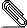# Re: [HACKERS] PoC: Partial sort

```On Tue, Feb 11, 2014 at 7:59 AM, Alexander Korotkov <aekorot...@gmail.com>
wrote:
>
> Done. Patch is splitted.
>```
```
I've started to look at this, and for now I'm still finding my way around
the patch, so I'm not quite there yet with understanding everything.
Never-the-less it seems best to post my comments early, so as to help
maintain concurrency between the review and getting the patch into shape.

I've only been looking at partial-sort-basic-1.patch so far;

The patch no longer applies to master, but this was only due to a tab being
replaced by 2 spaces in a pgident run. I've attached an updated patch which
currently applies without any issues.

Here's a few notes from reading over the code:

* pathkeys.c

EquivalenceMember *member = (EquivalenceMember *)

occurs in costsize.c

* pathkeys.c

The following fragment:

n = pathkeys_common(root->query_pathkeys, pathkeys);

if (n != 0)
{
/* It's useful ... or at least the first N keys are */
return n;
}

return 0; /* path ordering not useful */
}

/* return the number of path keys in common, or 0 if there are none */
return pathkeys_common(root->query_pathkeys, pathkeys);

* execnodes.h

In struct SortState, some new fields don't have a comment.

I've also thrown a few different workloads at the patch and I'm very
impressed with most of the results. Especially when LIMIT is used, however
I've found a regression case which I thought I should highlight, but for
now I can't quite see what could be done to fix it.

create table a (x int not null, y int not null);
insert into a select x.x,y.y from generate_series(1,1000000) x(x) cross
join generate_series(1,10) y(y);

Patched:
explain analyze select x,y from a where x+0=1 order by x,y limit 10;
QUERY PLAN
------------------------------------------------------------------------------------------------------------------------------------
Limit  (cost=92.42..163.21 rows=10 width=8) (actual
time=6239.426..6239.429 rows=10 loops=1)
->  Partial sort  (cost=92.42..354064.37 rows=50000 width=8) (actual
time=6239.406..6239.407 rows=10 loops=1)
Sort Key: x, y
Presorted Key: x
Sort Method: quicksort  Memory: 25kB
->  Index Scan using a_x_idx on a  (cost=0.44..353939.13
rows=50000 width=8) (actual time=0.059..6239.319 rows=10 loops=1)
Filter: ((x + 0) = 1)
Rows Removed by Filter: 9999990
Planning time: 0.212 ms
Execution time: 6239.505 ms
(10 rows)

Time: 6241.220 ms

Unpatched:
explain analyze select x,y from a where x+0=1 order by x,y limit 10;
QUERY PLAN
--------------------------------------------------------------------------------------------------------------------
Limit  (cost=195328.26..195328.28 rows=10 width=8) (actual
time=3077.759..3077.761 rows=10 loops=1)
->  Sort  (cost=195328.26..195453.26 rows=50000 width=8) (actual
time=3077.757..3077.757 rows=10 loops=1)
Sort Key: x, y
Sort Method: quicksort  Memory: 25kB
->  Seq Scan on a  (cost=0.00..194247.77 rows=50000 width=8)
(actual time=0.018..3077.705 rows=10 loops=1)
Filter: ((x + 0) = 1)
Rows Removed by Filter: 9999990
Planning time: 0.510 ms
Execution time: 3077.837 ms
(9 rows)

Time: 3080.201 ms

As you can see, the patched version performs an index scan in order to get
the partially sorted results, but it does end up quite a bit slower than
the seqscan/sort that the unpatched master performs. I'm not quite sure how
realistic the x+0 = 1 WHERE clause is, but perhaps the same would happen if
something like x+y = 1 was performed too.... After a bit more analysis on
this, I see that if I change the 50k estimate to 10 in the debugger that
the num_groups is properly estimated at 1 and it then performs the seq scan
instead. So it looks like the costings of the patch are not to blame here.
(The 50k row estimate comes from rel tuples  / DEFAULT_NUM_DISTINCT)

That's all I have at the moment... More to follow soon.

Regards

David Rowley
```partial-sort-basic-1_rebased.patch
Description: Binary data

```--
Sent via pgsql-hackers mailing list (pgsql-hackers@postgresql.org)
To make changes to your subscription:
http://www.postgresql.org/mailpref/pgsql-hackers
```